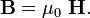# Magnetic constant

The magnetic constant μ0 (also known as vacuum permeability or permeability of free space) is a universal physical constant, relating mechanical and electromagnetic units of measurement. In the International System of Units (SI), its value is exactly expressed by:

μ0 = 4π × 10−7 N/A2 = 4π×10−7 henry/metre (H/m) , or approximately 1.2566×10−6 H/m.

This value is a consequence of the definition of the ampere in terms of forces between wires, see Ampère's equation. In vacuum, the magnetic constant is the ratio of the magnetic B-field (entering the expression for the Lorentz force) to the magnetic H-field (the field inside a solenoid):$\mathbf{B} = \mu_0 \ \mathbf{H}.$

In SI units the magnetic constant μ0 is related to the electric constant ε0 and to the speed of light in vacuum by c ² ε0 μ0 = 1.

In Gaussian units, the symbols μ0 and ε0 do not appear. Also, in Gaussian units, the speed of light is a measured, not a defined quantity.

## Terminology

Historically, the constant μ0 has had different names. A now rather obsolete term is "magnetic permittivity of vacuum". In the 1987 IUPAP Red book this constant was called permeability of vacuum. Currently the nomenclature in physics is magnetic constant. The permeability μ ≡ μr μ0 is equal to μ0 for the vacuum, i.e., for the vacuum the relative permeability μr = 1.

##  Footnotes

1. 1.0 1.1 Magnetic constant. 2006 CODATA recommended values. NIST. Retrieved on 2007-08-08.
2. Unit of electric current (ampere). Historical context of the SI. NIST. Retrieved on 2007-08-11.
3. Markus Reiher, Alexander Wolf (2009). Relativistic quantum chemistry: the fundamental theory of molecular science. Wiley-VCH, p. 7. ISBN 3527312927.
4.  ; (the IUPAP "Red book").
5. National Physical Laboratory, UK (1998). Fundamental Physical Constants p. 2.